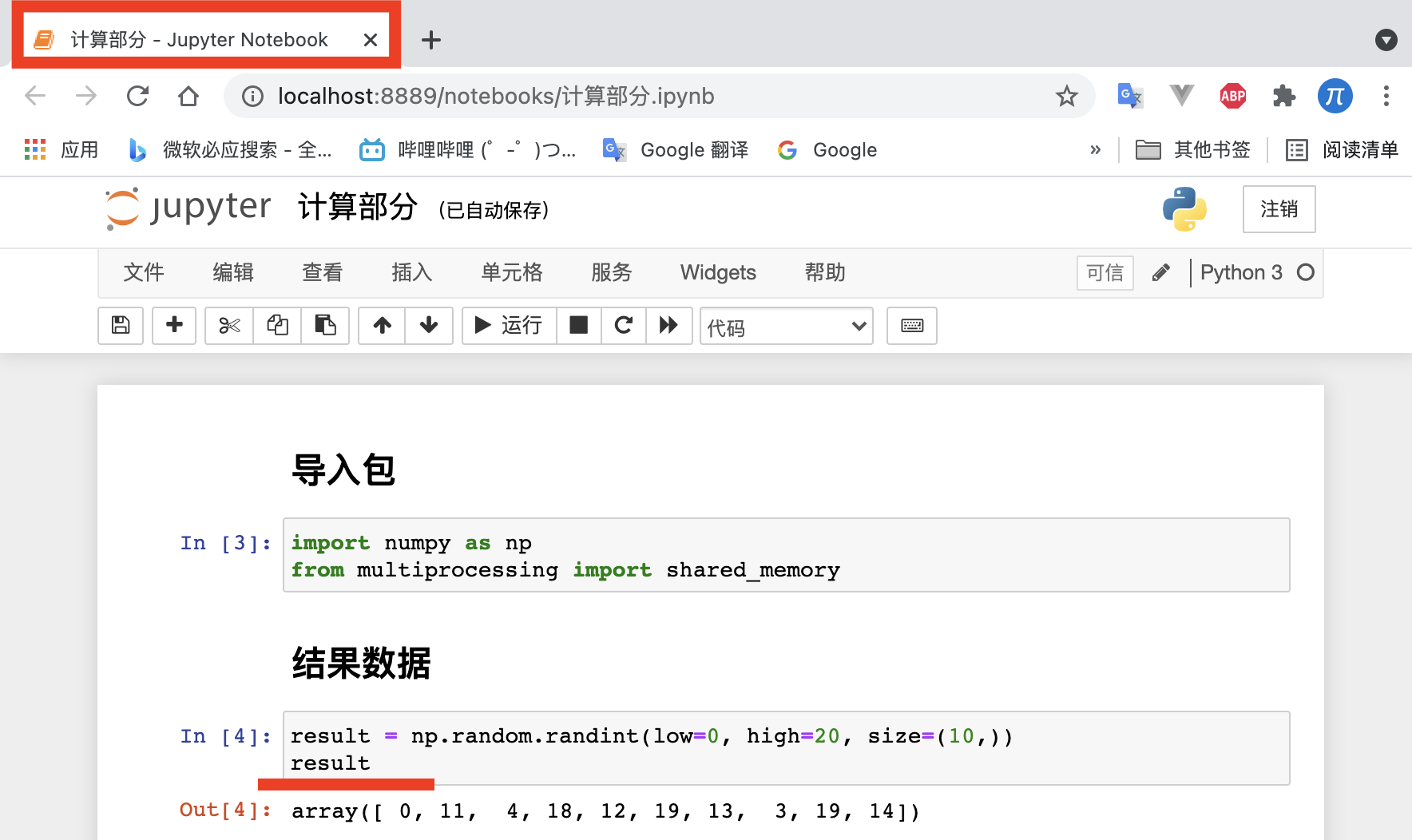墨滴yuanzhoulvpi

2021/09/11  阅读：50  主题：橙心

# jupyter还能这么用(连个鬼jupyter怎么共享数据）

## 开始尝试

1. 第一个jupyter notebook名称叫【计算部分】，这里面有个result，是我们需要的非常重要的结果。2. 第二个jupyter notebook名称叫【使用部分】，这个是使用【计算部分】的result。

## 步骤

1. 先在【计算部分】里面加上这些代码。
``class ShareData(object):    def __init__(self, data):        self.data = data        self.shm = shared_memory.SharedMemory(create=True, size=self.data.nbytes)        def run(self):        self.tempdata = np.ndarray(self.data.shape, dtype=self.data.dtype, buffer=self.shm.buf)        self.tempdata[:] = self.data[:]        print(f"数据储存的位置为：{self.shm.name}")        print(f"数据的大小尺寸为： {self.data.shape}")        print(f"数据的类型为: {self.data.dtype}")# sharedata = ShareData(data=result)sharedata.run()``

1. 在【使用部分】这样写代码： 需要注意的是：在【计算部分】中的【数据储存位置】、【数据的大小】、【数据的类型】三个参数，都放到【使用部分】里面。这样写代码：
``import numpy as np from multiprocessing import shared_memory# def returndata(name, shape, dtype):#     exist_shm = shared_memory.SharedMemory(name=name)#     data = np.ndarray(shape=shape,dtype=dtype,buffer=exist_shm.buf)#     return data# getdata = returndata(name='psm_10d26010', shape=(10,), dtype=np.int64)# getdataexist_shm = shared_memory.SharedMemory(name='psm_6d2ee752')data = np.ndarray(shape=(10,),dtype=np.int64,buffer=exist_shm.buf)data``

1. 然后就可以看到，【使用部分】的【data】数据是和【计算部分】的result是一模一样的。

## 工作原理：

SharedMemory创建一个新的共享内存块或者连接到一片已经存在的共享内存块。每个共享内存块都被指定了一个全局唯一的名称。通过这种方式，进程可以使用一个特定的名字创建共享内存区块，然后其他进程使用同样的名字连接到这个共享内存块。以下示例展示了一个现实中的例子，使用 SharedMemory 类和 NumPy arrays 结合, 从两个 Python shell 中访问同一个 numpy.ndarray 。yuanzhoulvpi

2021/09/11  阅读：50  主题：橙心